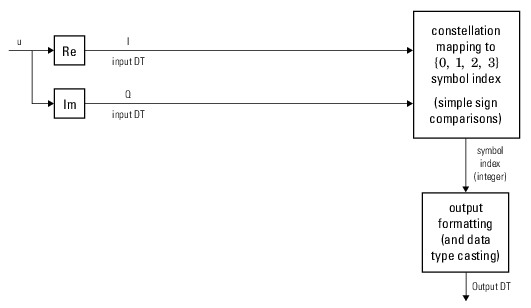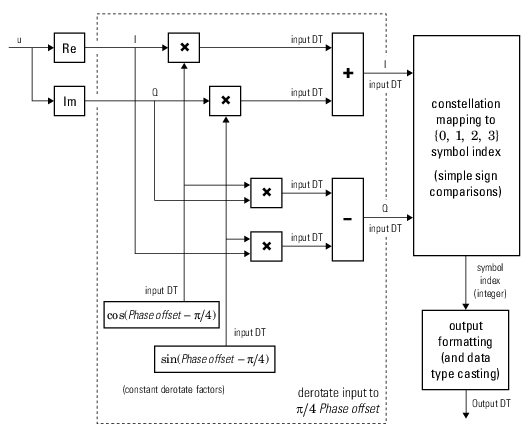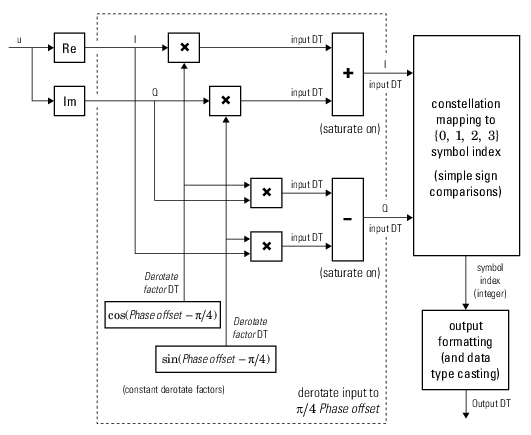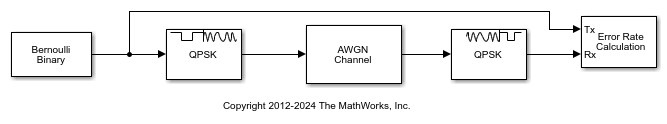# QPSK Demodulator Baseband

Demodulate QPSK-modulated data

## Library

PM, in Digital Baseband sublibrary of Modulation

•## Description

The QPSK Demodulator Baseband block demodulates a signal that was modulated using the quadrature phase shift keying method. The input is a baseband representation of the modulated signal.

The input must be a complex signal. This block accepts a scalar or column vector input signal. For information about the data types each block port supports, see Supported Data Types.

### Algorithm

Hard-Decision QPSK Demodulator Signal Diagram for Trivial Phase Offset (odd multiple of π/4)Hard-Decision QPSK Demodulator Floating-Point Signal Diagram for Nontrivial Phase OffsetHard-Decision QPSK Demodulator Fixed-Point Signal Diagram for Nontrivial Phase OffsetThe exact LLR and approximate LLR cases (soft-decision) are described in Exact LLR Algorithm and Approximate LLR Algorithm.

## Dialog BoxThe phase of the zeroth point of the signal constellation.

Constellation ordering

Determines how the block maps each integer to a pair of output bits.

Output type

Determines whether the output consists of integers or bits.

If the Output type parameter is set to `Integer` and Constellation ordering is set to `Binary`, then the block maps the point

exp(jθ + jπm/2)

to m, where θ is the Phase offset parameter and m is 0, 1, 2, or 3.

The reference page for the QPSK Modulator Baseband block shows the signal constellations for the cases when Constellation ordering is set to either `Binary` or `Gray`.

If the Output type is set to `Bit`, then the output contains pairs of binary values if Decision type is set to `Hard decision`. The most significant bit (i.e. the left-most bit in the vector), is the first bit the block outputs.

If the Decision type is set to `Log-likelihood ratio` or ```Approximate log-likelihood ratio```, then the output contains bitwise LLR or approximate LLR values, respectively.

Decision type

Specifies the use of hard decision, LLR, or approximate LLR during demodulation. This parameter appears when you select `Bit` from the Output type drop-down list. The output values for Log-likelihood ratio and Approximate log-likelihood ratio decision types are of the same data type as the input values. For integer output, the block always performs Hard decision demodulation.

See Exact LLR Algorithm and Approximate LLR Algorithm for algorithm details.

Noise variance source

This field appears when ```Approximate log-likelihood ratio``` or `Log-likelihood ratio` is selected for Decision type.

When set to `Dialog`, the noise variance can be specified in the Noise variance field. When set to `Port`, a port appears on the block through which the noise variance can be input.

Noise variance

This parameter appears when the Noise variance source is set to `Dialog` and specifies the noise variance in the input signal. This parameter is tunable in normal mode, Accelerator mode and Rapid Accelerator mode.

If you use the Simulink® Coder™ rapid simulation (RSIM) target to build an RSIM executable, then you can tune the parameter without recompiling the model. This is useful for Monte Carlo simulations in which you run the simulation multiple times (perhaps on multiple computers) with different amounts of noise.

The LLR algorithm involves computing exponentials of very large or very small numbers using finite precision arithmetic and would yield:

• `Inf` to `-Inf` if Noise variance is very high

• `NaN` if Noise variance and signal power are both very small

In such cases, use approximate LLR, as its algorithm does not involve computing exponentials.

Data Types Pane for Hard-DecisionOutput

For bit outputs, when Decision type is set to `Hard decision`, the output data type can be set to `'Inherit via internal rule'`, ```'Smallest unsigned integer'```, `double`, `single`, `int8`, `uint8`, `int16`, `uint16`, `int32`, `uint32`, or `boolean`.

For integer outputs, the output data type can be set to ```'Inherit via internal rule'```, ```'Smallest unsigned integer'```, `double`, `single`, `int8`, `uint8`, `int16`, `uint16`, `int32`, or `uint32`.

When this parameter is set to ```'Inherit via internal rule'``` (default setting), the block will inherit the output data type from the input port. The output data type will be the same as the input data type if the input is a floating-point type (`single` or `double`). If the input data type is fixed-point, the output data type will work as if this parameter is set to `'Smallest unsigned integer'`.

When this parameter is set to ```'Smallest unsigned integer'```, the output data type is selected based on the settings used in the Hardware Implementation pane of the Configuration Parameters dialog box of the model.

If `ASIC/FPGA` is selected in the Hardware Implementation pane, and Output type is `Bit`, the output data type is the ideal minimum one-bit size, i.e., `ufix(1)`. For all other selections, it is an unsigned integer with the smallest available word length large enough to fit one bit, usually corresponding to the size of a char (e.g., `uint8`).

If `ASIC/FPGA` is selected in the Hardware Implementation pane, and Output type is `Integer`, the output data type is the ideal minimum two-bit size, i.e., `ufix(2)`. For all other selections, it is an unsigned integer with the smallest available word length large enough to fit two bits, usually corresponding to the size of a char (e.g., `uint8`).

Derotate factor

This parameter only applies when the input is fixed-point and Phase offset is not an even multiple of π/4.

You can select `Same word length as input` or `Specify word length`, in which case you define the word length using an input field.

Data Types Pane for Soft-DecisionFor bit outputs, when Decision type is set to `Log-likelihood ratio` or ```Approximate log-likelihood ratio```, the output data type is inherited from the input (e.g., if the input is of data type `double`, the output is also of data type `double`).

## Examples

expand all

Modulate and demodulate a noisy QPSK signal.

Use the Open model button to open the QPSK demodulation model.Run the simulation. The results are saved to the base workspace, where the variable `ErrorVec` is a 1-by-3 row vector. The BER is found in the first element.

Display the error statistics. For the Eb/No provided, 4.3 dB, the resultant BER is approximately 0.01. Your results may vary slightly.

```ans = 0.0112 ```

Increase the Eb/No to 7 dB. Rerun the simulation, and observe that the BER has decreased.

```ans = 1.0000e-03 ```

## Supported Data Types

PortSupported Data Types

Input

• Double-precision floating point

• Single-precision floating point

• Signed fixed-point when:

• Output type is `Integer`

• Output type is `Bit` and Decision type is `Hard-decision`

Var

• Double-precision floating point

• Single-precision floating point

Output

• Double-precision floating point

• Single-precision floating point

• Boolean when Output type is `Bit` and Decision type is `Hard-decision`

• 8-, 16-, 32- bit signed integers

• 8-, 16-, 32- bit unsigned integers

• ufix(1) in ASIC/FPGA when Output type is `Bit`

• ufix(2) in ASIC/FPGA when Output type is `Integer`

## Pair Block

QPSK Modulator Baseband

## Version History

Introduced before R2006a